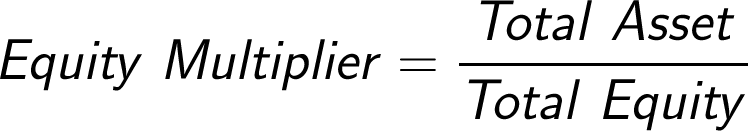# Equity Multiplier Formula

The Equity Multiplier Formula is an accounting formula used to indicate the level of financial leverage by a company. The formula for the equity multiplier is total assets divided by stockholder’s equity.